SSC  >  Thermodynamics - 3

# Thermodynamics - 3

Test Description

## 20 Questions MCQ Test Mock Test Series for SSC JE Mechanical Engineering | Thermodynamics - 3

Thermodynamics - 3 for SSC 2022 is part of Mock Test Series for SSC JE Mechanical Engineering preparation. The Thermodynamics - 3 questions and answers have been prepared according to the SSC exam syllabus.The Thermodynamics - 3 MCQs are made for SSC 2022 Exam. Find important definitions, questions, notes, meanings, examples, exercises, MCQs and online tests for Thermodynamics - 3 below.
Solutions of Thermodynamics - 3 questions in English are available as part of our Mock Test Series for SSC JE Mechanical Engineering for SSC & Thermodynamics - 3 solutions in Hindi for Mock Test Series for SSC JE Mechanical Engineering course. Download more important topics, notes, lectures and mock test series for SSC Exam by signing up for free. Attempt Thermodynamics - 3 | 20 questions in 12 minutes | Mock test for SSC preparation | Free important questions MCQ to study Mock Test Series for SSC JE Mechanical Engineering for SSC Exam | Download free PDF with solutions
 1 Crore+ students have signed up on EduRev. Have you?
Thermodynamics - 3 - Question 1

### In a P-V diagram for pure substance, the constant temperature lines in saturated liquid-vapour region are ________.

Thermodynamics - 3 - Question 2

### At critical temperature the _______.

Detailed Solution for Thermodynamics - 3 - Question 2

The critical temperature is the highest temperature at which vapour and liquid phases can coexist in equilibrium. For gases, the critical temperature is the one above which a gas cannot be liquefied regardless of the pressure applied. Also, note that dryness fraction is not defined at the critical point

Thermodynamics - 3 - Question 3

### If the entropy of the universe decreases. What this depicts about the nature of the process?

Detailed Solution for Thermodynamics - 3 - Question 3

The universe is formed by system and surrounding and considered as an isolated system.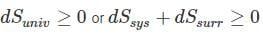i) The energy of the world (universe) is constant.

ii) The entropy of the world tends towards a maximum.

iii) The entropy increase of an isolated system is a measure of the extent of irreversibility of the process undergone by the system.

In actual practice the reversible isentropic process never really occurs, it is only an ideal process. In actual practice whenever there is a change in the state of the system the entropy of the system increases. The entropy of the universe increases because energy never flows uphill spontaneously.

If in any process, if the entropy of the universe decreases, then the process will be an impossible process.

Thermodynamics - 3 - Question 4

Which of the following properties are NOT sufficient to determine the properties of a vapour?

1. Temperature
2. Pressure
3. Dryness fraction
4. Specific volume
Detailed Solution for Thermodynamics - 3 - Question 4

In properties of vapour, we require at least two independent properties to determine the state of the vapour. Both pressure and temperature are dependent properties so, if we know only pressure and temperature, we can’t determine the properties of vapour.

Thermodynamics - 3 - Question 5

If the ratio of the lower to the higher absolute temperature is 7/8, then what will be the COP of the Carnot refrigerator?

Detailed Solution for Thermodynamics - 3 - Question 5

The Carnot COP of a refrigerator working between the temperature limit of TH and TL is given as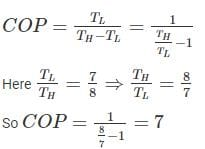Kindly note that the COP of Carnot heat pump or refrigerator depends only on the upper and lower temperature limits and COP is independent of the working fluid.

Thermodynamics - 3 - Question 6

One kg of air (R=287 J/kg-K) goes through an irreversible process between two equilibrium state 1 (30°C, 1.2 m3) and state 2 (30°C, 0.8 m3). What is the change in entropy (in J/kg-K)?

Detailed Solution for Thermodynamics - 3 - Question 6

Change of entropy in a reversible process: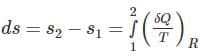Entropy changes for a closed system: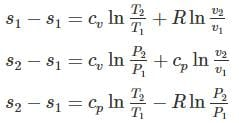For constant volume: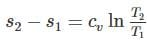For constant pressure: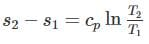For constant temperature process: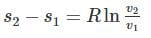For adiabatic process (Reversible):

dQ = 0 ⇒ dS = 0

Calculation:

Here T1 = T2

So the process is isothermal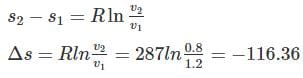Thermodynamics - 3 - Question 7

In a surrounding, the amount of irreversibility of a process undergone by a system is determined by ________.

Detailed Solution for Thermodynamics - 3 - Question 7

The universe is formed by system and surrounding and considered as an isolated system. The entropy of the universe always tends towards a maximum. The entropy increase of an isolated system is a measure of the extent of irreversibility of the process undergone by the system.

Thermodynamics - 3 - Question 8

Clausius’ statement and Kelvin - Planck’s statement are ________.

Detailed Solution for Thermodynamics - 3 - Question 8

There are two statements of second law of thermodynamics.

Clausius statement: No device can operate on a cycle and produce effect that is solely the heat transfer from a lower-temperature body to a higher-temperature body

Kelvin Plank statement: It is impossible for any device that operates on a cycle to receive heat from a single reservoir and produce a net amount of work

Equivalence of two statements: If some device violates one statement, it also violates the other statement, and vice versa.

Thermodynamics - 3 - Question 9

A Carnot engine operates between temperature 1000 K and 400 K. The heat rejected by the first Carnot engine is used by the second Carnot engine, whose sink temperature is 200 K. If the net heat absorbed by the first Carnot engine is 200 MJ. What is the heat rejected (in MJ) by the second Carnot engine?

Thermodynamics - 3 - Question 10

In a general compression process, 2 kJ of mechanical work is supplied to 4 kg of fluid and 800 J of heat is rejected to the cooling jacket. The change in specific internal energy would be

Detailed Solution for Thermodynamics - 3 - Question 10

From the first law of thermodynamics

dQ = dU + dW

dQ = -800 J (As the heat is rejected from the system)

dW = -2000 J (as work is supplied to system)

Change in internal energy = dQ – dW = -800 – (-2000) = 1200 J

Change in specific internal energy = 1200/4 = 300 J/kg  (mass is 4 kg)

Thermodynamics - 3 - Question 11

Which of the below stated are properties of a PMM-2?

1. When the net work is equal to the heat absorbed and work efficiency is 100%.
2. Heat is exchanged from one heat reservoir only.
3. It violates Kelvin-Planck statement.
4. It is a hypothetical machine
Detailed Solution for Thermodynamics - 3 - Question 11

Kelvin – Planck statement states “it is impossible to construct an engine, which is operating in a cycle produces no other effect except to external heat from a single reservoir and do equivalent amount of work.

A PMM II is the heat engine which produces the work equal to the heat supplied. Thus, it violates the second law of thermodynamics and It is a hypothetical machine.

On the other hand, Perpetual motion machine of type-I violates the first law of thermodynamics.

Thermodynamics - 3 - Question 12

A gas having a negative Joule-Thomson coefficient (μJ < 0), when throttled, will

Detailed Solution for Thermodynamics - 3 - Question 12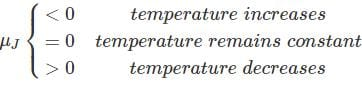Thermodynamics - 3 - Question 13

According to which law, all perfect gases change in volume by (1/273)th of their original volume at 0°C for every 1°C change in temperature when pressure remains constant

Detailed Solution for Thermodynamics - 3 - Question 13

temperature, when the absolute pressure remains constant." Mathematically,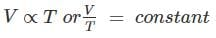Or “All perfect gases change in volume by 1 / 273 th of its original volume at 0° C for every 1° C change in temperature, when the pressure remains constant."

Boyles law: The absolute pressure of a given mass of a perfect gas varies inversely as its volume, when the temperature remains constant." Mathematically,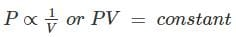Thermodynamics - 3 - Question 14

For a single component three phase mixture, the number of independent variable properties are

Detailed Solution for Thermodynamics - 3 - Question 14

As per Gibb’s phase rule,

F = C – P + 2

Where F = Degree of freedom

C = Number of chemical components

P = No of Phases

F = 1 – 3 + 2 = 0

Thermodynamics - 3 - Question 15

Three states of matter are distinguished with respect to molecules by the ______.

Detailed Solution for Thermodynamics - 3 - Question 15

Matter around us exists in three different states – solid, liquid and gas. They are distinguished by their intermolecular attraction force, molecular arrangement and the mobility of the system.

Particles in a:

• Gas vibrate and move freely at high speeds.
• Liquid vibrate, move about, and slide past each other.
• Solid vibrate (jiggle) but generally do not move from place to place.
Thermodynamics - 3 - Question 16

The triple point on a P - V diagram is ________.

Thermodynamics - 3 - Question 17

An automobile heats up while lying in a parking lot on a sunny day. The process can be assumed to be

Detailed Solution for Thermodynamics - 3 - Question 17

The process of heating up of an automobile can be assumed as isobaric. Because the pressure inside the car is constant. So out of given options the option b is correct.

Thermodynamics - 3 - Question 18

While working between temperatures 150 K and 300 K, the entropy change experienced by the Carnot engine during heat addition is 1 kJ/K, the work produced (kJ) by the engine is ________.

Thermodynamics - 3 - Question 19

In free expansion process

Detailed Solution for Thermodynamics - 3 - Question 19

No heat interaction takes place

Hence dQ = 0

From first law

dQ = du + dW

du = 0

Thermodynamics - 3 - Question 20

There is fixed mass and energy in

Detailed Solution for Thermodynamics - 3 - Question 20

Closed System: There is no mass transfer across the system boundary but energy transfer takes place into or out of the system.

Open System: In this type of system both mass and energy transfer takes place across the boundary of system.

Isolated System: In this type of system neither mass nor energy crosses the boundary of the system. So it is a system with fixed mass and fixed energy.

## Mock Test Series for SSC JE Mechanical Engineering

3 videos|1 docs|55 tests
 Use Code STAYHOME200 and get INR 200 additional OFF Use Coupon Code
Information about Thermodynamics - 3 Page
In this test you can find the Exam questions for Thermodynamics - 3 solved & explained in the simplest way possible. Besides giving Questions and answers for Thermodynamics - 3, EduRev gives you an ample number of Online tests for practice

## Mock Test Series for SSC JE Mechanical Engineering

3 videos|1 docs|55 tests